Next: 8.2.1 Printing of a Up: 8. Simulation Examples Previous: 8.1.2 Imaging Enhancement Techniques

# 8.2 Photoresist Exposure and Development Simulation

This application is a comprehensive study of DUV printing of a single contact hole over planar and stepped topographies. Three different illumination apertures are investigated whether they can provide a greater depth of focus. The presented simulation results clearly demonstrate the capability of the differential method for rigorous, nonplanar, three-dimensional lithography simulation. The severe impact of a reflective nonplanarity--in our case it is a-silicon but any metal behaves similarly--is correctly reproduced, which is of great interest for the process engineer since printing over nonplanar reflective substrates becomes increasingly difficult due to the growing complexity and package density of the semiconductor devices.

In all cases the simulation domain is 1.0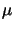m x 1.0m x 0.7m large, and the substrate material is silicon with a refractive index of nSi = 1.68 + j3.58. For the nonplanar simulations a step with a height of 0.25m and a slope of 45o is assumed to be located in the middle of the simulation domain. The step material is either dielectric oxide with a refractive index of nSiO2 = 1.508 or reflective a-silicon with a refractive index of na-Si = 1.69 + j2.76. A non-bleaching DUV resist is chosen with a refractive index of nResist = 1.65 + j0.02. All material parameters are taken from Table I of . The mask is situated in the center of the geometry, i.e., exactly above the slope, and the mask-opening is 0.25m x 0.25m wide. A sketch of the investigated configuration is shown in Figure 8.16.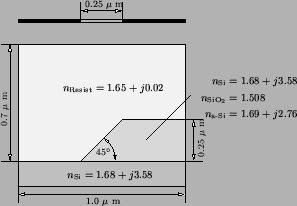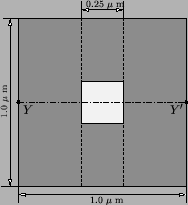The stepper is a DUV projection printing system with a wavelength of 248 nm and a fixed numerical aperture of NA = 0.5. Three different illumination apertures are compared, namely coherent, partially coherent or circular with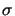= 0.8, and quadrupole with= 0.1 and X = Y = 0.7. The number and location of the source points are illustrated in the discretized wavevector diagram of Figure 8.17. The exposure dose is set to 120 mJ/cm2 and the development time is chosen so that in all cases the full contact is opened. For the resist an absorption parameter of C = 0.013 cm2/mJ is assumed, and Kim's R'-model is used to calculate the development rate from the PAC concentration (cf. Section 7.2.1). The R'-parameter set is taken from Table IV of : R1 = 0.25m/s, R2 = 0.0005m/s, and R3 = 7.4. All simulations are performed with ideal focus as well as with a defocus of 1m above the resist surface.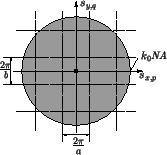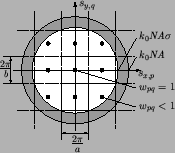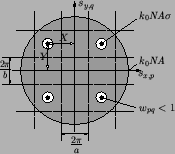For the simulation of the EM field propagation inside the simulation domain 51 Fourier modes in x-direction and 21 in y-direction are used to represent the EM field. These values correspond to cut-off frequencies of Nx = 25 and Ny = 10 applied to the differential method (cf. Section 6.2.5). The differential method thus consumes approximately 300 MB memory (cf. Section 6.5.1). The number Nz of discretization points is in the planar and dielectric cases 50, and in the reflective case 75 since the stronger absorption in a-silicon has to be resolved properly to obtain a stable performance of the stabilized march algorithm. The run-times range between 4 to 5 hours on a DEC-600/333 workstation depending on the step material. The development simulation is performed with a cell density of 300 cells/m. The memory usage is 20 MB assuming 1 Byte per cell and the run-time is 25 minutes.

In case of a planar topography standing waves are caused by reflections at the substrate interface due to the abrupt change of the refractive index. This well-known standing wave phenomenon results in three dimensions into ideally oval contours of the PAC concentrations and, correspondingly, of the developed resist profiles (cf. Figure 8.18 to Figure 8.20). The strict regularity is due to the constant optical thickness across the whole simulation area. The situation changes for the nonplanar cases since the optical thickness varies. The contours are less regular, whereby the degree of distortion depends on the underlying step material (cf. Figure 8.21 to Figure 8.26). A comparison of the two simulated nonplanar topographies exhibits a stronger impact of the reflective a-silicon step on the latent bulk images and developed resist profiles (cp. Figure 8.21 to Figure 8.23 with Figure 8.24 to Figure 8.26). The reason for the minor distortions caused by the dielectric step is that the real parts of the refractive indices of the oxide and the resist, Re{nSiO2} = 1.508 and Re{nResist} = 1.65, respectively, are almost matched and no reflections occur at the dielectric, i.e., Im{nSiO2} = 0.0 (cf. Figure 8.21 to Figure 8.23). In case of a-silicon the conductivity, i.e., the non-vanishing imaginary part of the refractive index Im{na-Si} = 2.76, forces a minimal node in the EM field distribution along the step surface, which results in a maximum of the PAC concentration within the exposed resist and thus in a minimum of the development rate (cf. Figure 8.24 to Figure 8.26). Furthermore, some fraction of the absorbed light intensity is scattered into nominally unexposed regions of the resist opposite the step resulting in an undesired development of these resist portions. This can be clearly seen in the developed resist profiles. For the same reasons as before this notching effect is more distinct for the reflective topography than for the dielectric (cp. Figure 8.21 to Figure 8.23 with Figure 8.24 to Figure 8.26).

Regarding the various illumination forms it can be seen that the circular and quadrupole apertures perform better in the defocus situation than the coherent. The difference is most pronounced in case of the planar substrate since no topography effects superpose the defocus phenomenon (cf. Figure 8.18 to Figure 8.20). The resolution for perfect focus, however, is almost equal for the three illumination forms. In addition to the three-dimensional plots the resist profiles are compared along the cut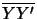going through the center of the contact hole (cf. Figure 8.16). In Figure 8.27 to Figure 8.29 the cross sections are shown for the three topographies. The diameter of the printed contact hole at the substrate surface can be extracted from the two-dimensional profiles. The results are summarized in Table 8.2.

Table 8.2: Contact hole diameters at the substrate surface for the planar, nonplanar dielectric, and nonplanar reflective topographies.

 Planar substrate dø [nm]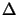f [m] conventional circular quadrupole 0.0 307 307 307 1.0 415 290 309
 Oxide step dø [nm]f [m] conventional circular quadrupole 0.0 272 272 272 1.0 281 272 272
 a-Silicon step dø [nm]f [m] conventional circular quadrupole 0.0 307 261 261 1.0 277 261 270

The simulations show the influence of a nonplanar topography on the overall lithographic performance. In general a reflective material, e.g., a conductor forming the interconnect between the individual devices, has a strong impact on the printing quality. For that reason chemical polishing was developed to avoid the strong nonplanarities occurring during the back-end process steps . In case of a dielectric material the effects are less pronounced. The formation of source/drain contacts is thus less problematic since the nonplanarity is a dielectric like oxide. Furthermore, the superior performance of advanced illumination apertures in case of focus errors is demonstrated. Since the simulated pitch was 1m all illumination apertures are capable to resolve the contact holes. However, in case of coherent illumination the opening diameter differs considerably between the focus and defocus situation and process latitude can not be matched. For that reason advanced illumination apertures are predominantly used in modern semiconductor lithography.Next: 8.2.1 Printing of a Up: 8. Simulation Examples Previous: 8.1.2 Imaging Enhancement Techniques
Heinrich Kirchauer, Institute for Microelectronics, TU Vienna
1998-04-17